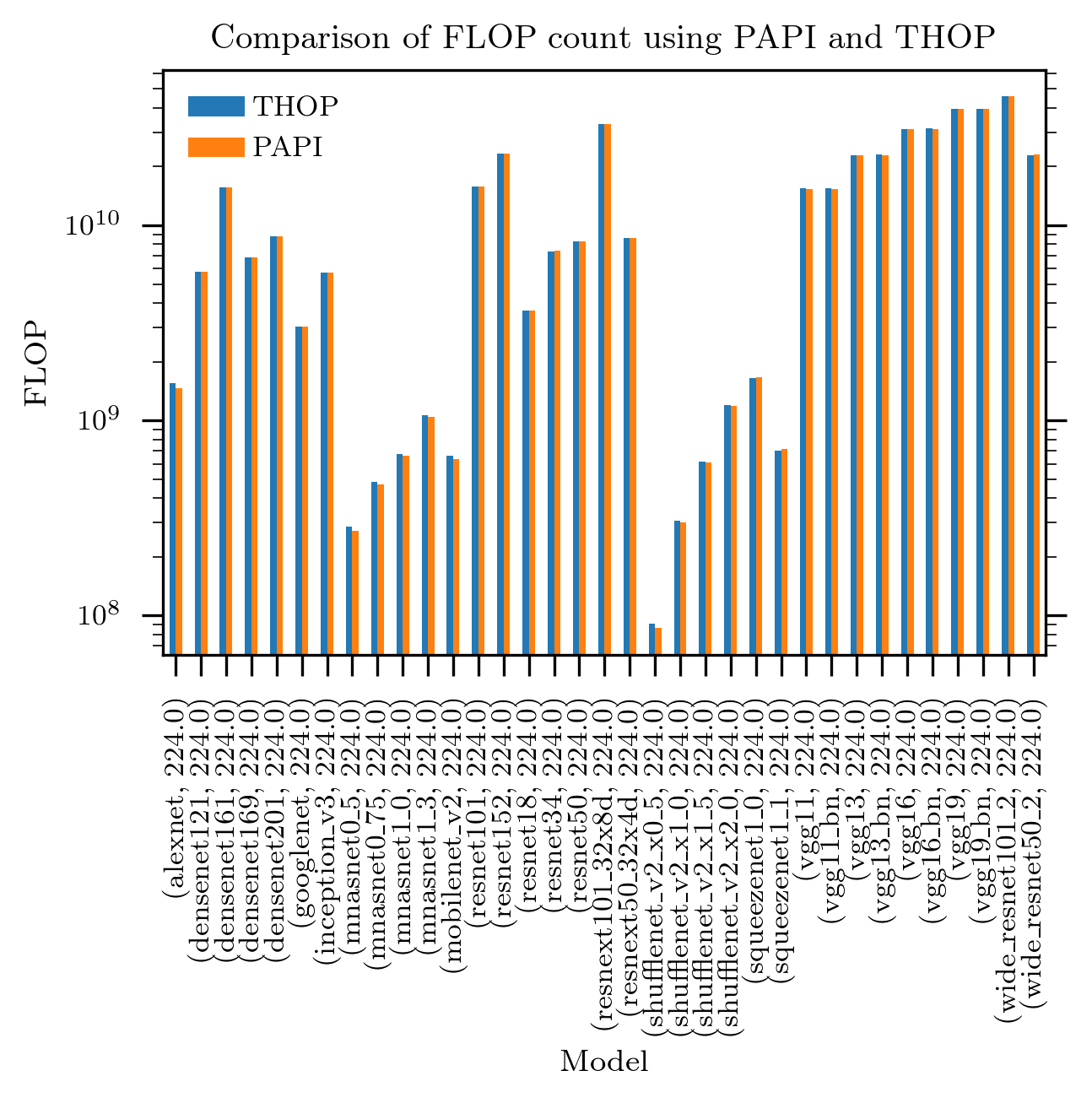PyTorch has a useful third-party module THOP which calculates the number of floating point (multiply/accumulate) operations needed to make an inference from a PyTorch neural network model. Here I compare THOP estimates of FLOPs to measurements made using CPU Performance monitors in order to cross-validate both techniques.

THOP works by having a registry of simple functions that predict the number of FLOPs needed for each stage of neural networks. The registry is pre-populated with following neural network stages:

``````    nn.Conv1d
nn.Conv2d
nn.Conv3d
nn.ConvTranspose1d
nn.ConvTranspose2d
nn.ConvTranspose3d
nn.BatchNorm1d
nn.BatchNorm2d
nn.BatchNorm3d
nn.ReLU
nn.ReLU6
nn.LeakyReLU
nn.MaxPool1d
nn.MaxPool2d
nn.MaxPool3d
nn.AvgPool1d
nn.AvgPool2d
nn.AvgPool3d
nn.Linear
nn.Dropout
nn.Upsample
nn.UpsamplingBilinear2d
nn.UpsamplingNearest2d
``````

Each function uses the dimensions of input data and any parameters controlling additional operations (e.g., `bias`) to estimate the operation count.

Here I compare the outputs of this way of estimating the FLOPs counts with an estimate made using CPU performance monitoring units, using the PAPI library, as described in this post.

The snippet of code which does this as follows:

``````
evl=["PAPI_DP_OPS"]
model_names = sorted(name for name in models.__dict__ if
name.islower() and not name.startswith("__") # and "inception" in name
and callable(models.__dict__[name]))
n=224
for name in model_names:
model = models.__dict__[name]().double()
dsize = (1, 3, n, n)
inputs = torch.randn(dsize, dtype=torch.float64)
high.start_counters([getattr(events, x) for x in evl])
total_ops, total_params = profile(model, (inputs,), verbose=False)
pmu=high.stop_counters()
#store results
``````

The basics are taken from the THOP benchmark library. The main things to note:

1. The neural network models are used in their double precision version, by calling the `.double()` method on the model. The reason for that is that the PAPI double precision counters are much better at accounting for vectorised instructions

2. The papi counter used is PAPI_DP_OPS, which counts the double-precision operations. This for the same as above, that this counter tracks the vectorised operations

3. THOP counts fused multiply/accumulate operations while PAPI counts individual operations. For this reason I multiply the THOP by a factor of 2 to compare to PAPI

The results of this experiment is shown below:It can be seen that the results of the two methods are very close, probably to within the margin of error of any practical further application of this.

## Discussion

The above results show that:

1. PAPI (with the python binding) is an easy way to get a reasonably accurate FLOP count estimate of an arbitrary (CPU) program, as long as double precision is used throughout

2. PAPI can be used to FLOP count of PyTorch models/programs that do not have the estimator functions for THOP

3. The results validate the THOP computations for all of these PyTorch models